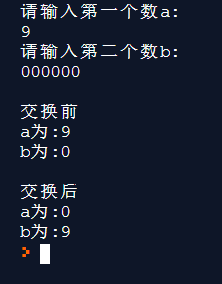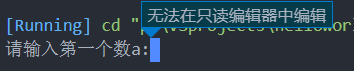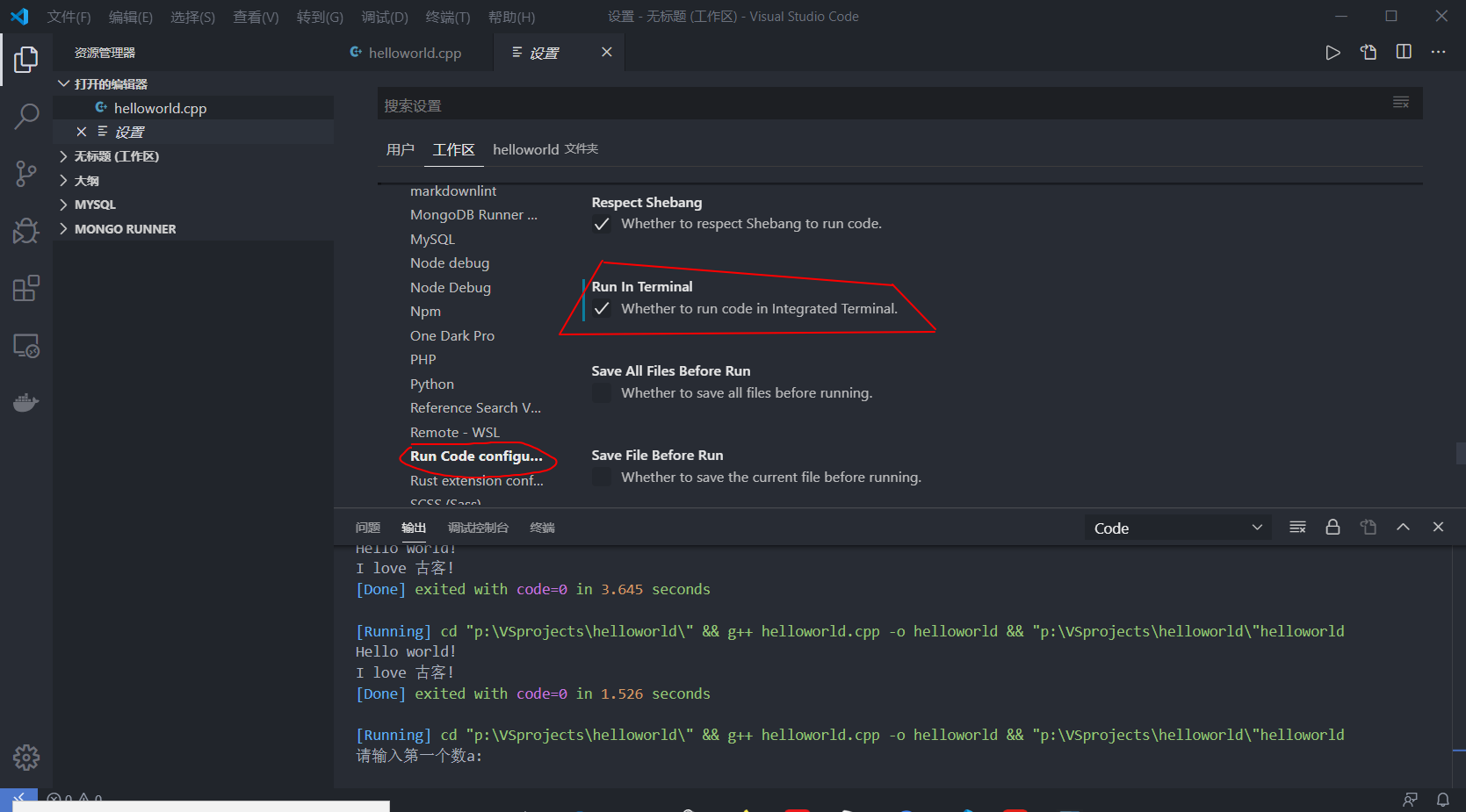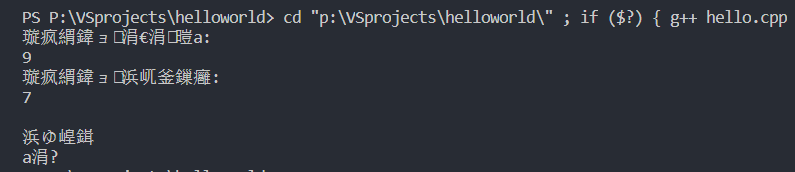# C++初学

## 1.准备开发环境

（更新：用Visual Studio了，因为弄了好久也没解决中文乱码的问题，哭了）

## 2.hello world详解

#include
using namespace std;

// main() 是程序开始执行的地方

int main()
{
cout << "Hello World"; // 输出 Hello World
return 0;  //因为主程序被声明为int数据类型，所以必须返回（return）一个值。
}
• #:告诉编辑器要预处理 include:表引入头文件 <iostream>:一个定义I/O流的文件，即输出流/输入流 (一般都要引入) 有两个引入方法：<> :编译环境的默认路径 ‘’：同文件夹内
• using namespace std：使用名为std的命名空间，此命名空间包含C++的基本功能 ;:终止语句符，表示一个逻辑表达式的结束，相当于中文的句号。（或用std::表示变量)
• //: 注释符号 /* */:此为多行注释
• Main函数是入口，程序是从int main()开始执行，无需参数，需返回整数，使用void main():无参数，不返回值
• 大括号{}：函数的主体，即函数的开始与结束
• cout:访问流对象 <<:输出右边的内容到显示器（不包含引号） >>:输出左边的
• return 0: 程序中的最后一条指令语句，终止main()函数，并返回0给调用的进程。非零值(通常为1)表示异常终止。(没有return也是可以运行的，但是程序大了会有不良影响，要养成好习惯)
• 其他补充：endl:换行符（也可以用/n) 区别：endl 时会刷新缓冲区，使得栈中的东西刷新一次，但用 “\n” 不会刷新，它只会换行，建议用endl

（备注：C++编译器是忽略空行和空格的，打代码时需注意可读性，方便维护，不要单单能运行就可以了）

#### 扩展使用：

##### 多行输出：
{
cout << "Hello world!" << endl;
cout << "I love 古客!";  //多行输出时，这个是正常都会运用的表达，但有更加方便的表达方法
return 0;
}
{
cout<<"Hello, world!\n"
<<"I love 古客!";
return 0;
}

#include<iostream>
#include<string>
using namespace std;
int main()
{
string str;
cin>>str;
cout<<str<<endl;
return 0;
}

（额，我也不是很懂，下次再补充吧，查了下，感觉要扩展很多知识，只能先放着了，看得懂英文的看一下这个

‘\0’ 产生空字符
‘\a’ 产生嘟声
‘\b’ 回退
‘\r’ 转到下一个定位点
‘\n’ 换行
‘\r’ 回车，跳到该行起点
‘\’’ 插入单引号
##### cin>>的用法：

cin可以连续从键盘读取想要的数据，以空格、tab或换行作为分隔符。实例程序如下。

#include <iostream>
using namespace std;

int main()
{
char a;
int b;
float c;
string
cin>>a>>b>>c;
cout<<a<<" "<<b<<" "<<c<<" "<<endl;

system("pause");
return 0;
}

（看到这个不知道有没有人跟我一样想到这个可以用来做游戏，像贪吃蛇，打飞机那样的小游戏，有时间可以试试(ノ￣▽￣)）

#include
#include
#include

using namespace std;

int main()
{
vector msg {"Hello", "C++", "World", "from", "VS Code", "and the C++ extension!"};   //

for (const string& word : msg)
{
cout << word << " ";
}
cout << endl;
}

vector:是向量类型，可以容纳许多类型的数据

string: 定义了一个变量类型、一个宏和各种操作字符数组的函数

C++ 中，分别使用.h 和.cpp 来定义一个类。

.h 中存放类的声明，函数原型（放在类的声明中）。

.cpp 存放函数体。

<> 先去系统目录中找头文件，如果没有在到当前目录下找。所以像标准的头文件 stdio.h、stdlib.h 等用这个方法。

“ “ 首先在当前目录下寻找，如果找不到，再到系统目录中寻找。 这个用于 include 自定义的头文件，让系统优先使用当前目录中定义的。

#### 可能出现的问题

##### 弹出窗口一闪而过，完全看不见输出了什么

include <cstdlib>
system("pause");  //在main函数里

## 3.各种数与变量的认识与使用

### 进制的转换

$(1001)_2$ $\Rightarrow$ $2^3+02^2+02^1+2^0$ =9

(原本还想写清楚一些，但是打数学公式实在是太麻烦了，相信大家一定懂了(*´ﾟ∀ﾟ｀)ﾉ )

Python版（这个我熟悉一些）

dec = int(input("输入十进制的数字:"))
print("十进制数为:",dec)
print("转换为二进制为：", bin(dec))
print("转换为八进制为：", oct(dec))
print("转换为十六进制为：", hex(dec))

C语言版

#### 十进制转换为八进制

#include <stdio.h>   //定义了三个变量类型、一些宏和各种函数来执行输入和输出
#include <math.h>   //引入数学计算

int convertDecimalToOctal(int decimalNumber);
int main()
{
int decimalNumber;

printf("输入一个十进制数: ");
scanf("%d", &decimalNumber);

printf("十进制数 %d 转换为八进制为 %d", decimalNumber, convertDecimalToOctal(decimalNumber));

return 0;
}

int convertDecimalToOctal(int decimalNumber)
{
int octalNumber = 0, i = 1;

while (decimalNumber != 0)
{
octalNumber += (decimalNumber % 8) * i;
decimalNumber /= 8;
i *= 10;
}

return octalNumber;
}

#### 八进制转换为十进制

#include <stdio.h>   //定义了三个变量类型、一些宏和各种函数来执行输入和输出
#include <math.h>

long long convertOctalToDecimal(int octalNumber);
int main()
{
int octalNumber;

printf("输入一个八进制数: ");
scanf("%d", &octalNumber);

printf("八进制数 %d  转换为十进制为 %lld", octalNumber, convertOctalToDecimal(octalNumber));

return 0;
}

long long convertOctalToDecimal(int octalNumber)
{
int decimalNumber = 0, i = 0;

while(octalNumber != 0)
{
decimalNumber += (octalNumber%10) * pow(8,i);
++i;
octalNumber/=10;
}

i = 1;

return decimalNumber;
}

（C语言我不太懂，这是菜鸟课程的）

## 4.一些性质和概念

### 运算符

#### 算术运算符

+ 把两个操作数相加 A + B 将得到 30
- 从第一个操作数中减去第二个操作数 A - B 将得到 -10
* 把两个操作数相乘 A * B 将得到 200
/ 分子除以分母 B / A 将得到 2
% 取模运算符，整除后的余数 B % A 将得到 0
++ 自增运算符，整数值增加 1 A++ 将得到 11

#include <iostream>
using namespace std;

int main()
{
int a = 21;
int c ;

// a 的值在赋值之前不会自增
c = a++;
cout << "Line 1 - Value of a++ is :" << c << endl ;

// 表达式计算之后，a 的值增加 1
cout << "Line 2 - Value of a is :" << a << endl ;

// a 的值在赋值之前自增
c = ++a;
cout << "Line 3 - Value of ++a is  :" << c << endl ;
return 0;
}

## 5.实例

### 输入输出与交互

#include

using namespace std;

int main()
{
int a ,b ,c;   //声明变量
cout << "请输入第一个数a:" << endl;
cin >> a;  //监视键盘输入值并赋值与a
cout << "请输入第二个数b:" << endl;
cin >> b;
cout << endl <<"交换前" << endl <<"a为:" << a << endl << "b为:" << b << endl;
c = a;
a = b;
b = c;

cout << endl <<"交换后"<< endl <<"a为:" << a << endl << "b为:" << b << endl;
}20200305230609

#### 可能出现的问题2020030518035220200305180852

VSCode终端其实调用的是cmd.exe，所以当这里出现中文乱码的时候要解决的是cmd的编码设置问题。2020030522085220200305221101

### 闰年的判断

#include <iostream>
using namespace std;
int main(){
int year;
bool lsLeapYear;

cout << "Enter the year: ";
cin>>year;
lsLeapYear = (year % 4 == 0 &&
year%100!=0)||(year%400==0);
if (lsLeapYear)
cout <<year <<" is a leap year" <<endl;
else
cout<<year<<" is not a leap year"<<endl;
return 0;
}

### 终极方案

https://c.runoob.com/compile/12 （推荐，对各种代码的应对措施较好）

https://tool.lu/coderunner/

https://repl.it/

https://www.bccn.net/run/（这个也有，是中文）

(吐槽下：C++的编译速度是真的慢，跟python没法比，而且用起来还好麻烦，需要想很多底层的事)

## 6.题目

### 2．试说明main()函数的功能。

main()是一个相当特殊的函数，代表着任何C++程序的进入点，也唯一且必须使用main作为函数名称。也就是说，当程序开始执行时，一定会先执行main()函数，而不管它在程序中的位置，编译程序都会找到它开始编译程序的内容，因此main()又称为“主函数”。

（VS家的可以）

8.编译阶段的主要工作是什么？

### 9.C++、Visual C++以及C++ Builder三者间的关系是什么？

C++是一种程序语言，Visual C++及C++ Builder则是分别由Microsoft与Borland公司所开发出来的支持C++的程序开发工具。

### 10.说明错误类型

（1）语法错误这是在程序开发过程中最常发生的错误。语法上的错误在程序编译时会发生编译时错误（compile time error），编译程序会将错误显示在“输出窗口”中，程序开发人员可以根据窗口上的提示迅速找出错误位置并加以修正。

（2）逻辑错误逻辑错误是程序中最难发现的臭虫（bug）。这类错误在编译时并不会出现任何错误信息，必须要依靠程序开发人员自行判断。这与程序开发人员的专业素养、经验和细心程度有着密不可分的关系。例如，薪资的计算公式、财务报表等，这些都必须在开发过程中以数据进行实际测试来确保日后程序运行结果的准确性和精确性。

## C++ 关键字

asm else new this
auto enum operator throw
bool explicit private true
break export protected try
case extern public typedef
catch false register typeid
char float reinterpret_cast typename
class for return union
const friend short unsigned
const_cast goto signed using
continue if sizeof virtual
default inline static void
delete int static_cast volatile
do long struct wchar_t
double mutable switch while
dynamic_cast namespace template

### 基本的内置类型

C++ 为程序员提供了种类丰富的内置数据类型和用户自定义的数据类型。下表列出了七种基本的 C++ 数据类型：

###### 参考链接：

https://www.runoob.com/cplusplus/cpp-basic-syntax.html

上一篇Matlab求定积分的部分方法

2020-03-03 古客python之正则表达式

2020-03-01 古客